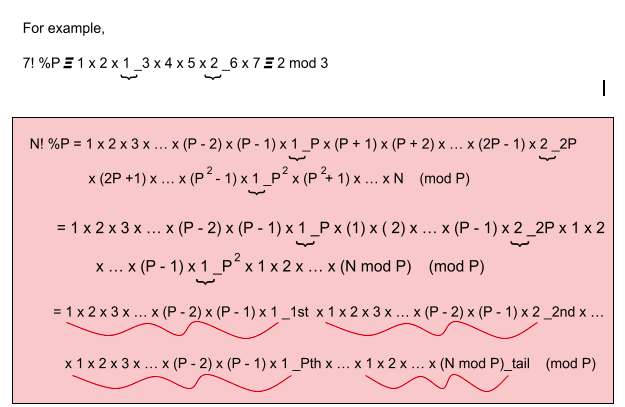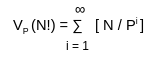# Factorial Modulo

### Introduction

In competitive programming, we often encounter the word modulo where we are asked to find out the answer in modulo as the answer can be huge, so we need to print out ‘modulo P’.

To find the factorial modulo, we must first compute ‘N!’ and then calculate ‘N! % P’. This solution works well when the value of ‘N!’ is very small. The value of  ‘N! % P’ is usually wanted for larger values of ‘N’ when ‘N!’ cannot fit into a variable and reasons an overflow. Therefore, calculation of ‘N!’ and then performing modulation is not a great idea to proceed as for slightly larger values of ‘N’ and ‘R’, there will always be an overflow where ‘N’ denotes the total number of items and ‘R’ represents the number of items chosen at a time, and ‘P’ denotes the prime number.CREDITS: GIPHY

### Working of factorial modulo

Complex formulas modulo some prime ‘P’, comprising factorials in both the numerator and denominator, such as those found in the formula for Binomial coefficients we come around often. We consider this situation when ‘P’ is relatively small.

Only when factorials exist in both the numerator and denominator of fractions, this problem make sense. Otherwise, the value of ‘P!’ and succeeding terms will be 0. In fractions, however, the factors of ‘P’ can be cancelled, resulting in a non-zero modulo ‘P’ statement.

Hence, we have the challenge to calculate ‘N! mod P’ without considering all of the numerous factors of ‘P’ that exist in the factorial. Let us write N’s prime factorization while removing all the ‘P’ factors and computing the product modulo ‘P’. This updated factorial is denoted by ‘N! % P’.### Code:-

#### Time Complexity:

The time complexity is O(N log log N).

{(N / 2) + (N / 3) + (N / 5) + (N / 7) + … + (N / P)},

where ‘P’ is the highest prime number, ‘P’ <= ‘N’

=> N {(1 / 2) + (1 / 3) + (1 / 5) + (1 / 7) + … + (1 / P)}

where {(1 / 2) + (1 / 3) + (1 / 5) + (1 / 7) + … + (1 / P)}

is the harmonic progression of sum of primes

=> N (log (log N))

as {(1 / 2) + (1 / 3) + (1 / 5) + (1 / 7) + … + (1 / P)} = log(log(N))

=> O(N log log N) time complexity

#### Space Complexity:

To find out the prime numbers from 1 to ‘N’, the space complexity is O(N) since we are creating an array of size ‘N’. It also means that if ‘N’ is constant, then the space complexity is O(1).

### Code:-

#### Time Complexity:

The time complexity for this approach will be O((P - N)* log N).

As, (P - 1)! = (P - 1) (P - 2) (P - 3) … 3 x 2 x 1

= (P - N) x (log N), where ’P’ is the prime number.

#### Space Complexity:

To find out the prime numbers from 1 to ‘N’, the space complexity is O(N) since we are creating an array of size ‘N’. It also means that if ‘N’ is constant, then the space complexity is O(1).

### Implementation of factorial modulo in C++

#### Time Complexity

The time complexity for this problem is O(N). As we are using one single iteration from 1 to ‘N’.

#### Space Complexity

The space complexity for this algorithm is O(1) as the space required by the above algorithm to process the data is constant.

What is meant by ‘ print in modulo 10+ 7 ’?

(10+ 7) is the first ten-digit prime number, and printing the answer in modulo ensures that the answer fits in the maximum value that our system can store, which is generally consistent with the question setter's code or case tester's code. This also makes sure that it prevents ‘integer overflow’ during the compilation process as it takes significant effort to store and process actual huge value.

What is the need for modulo?

We need modulo to avoid ‘integer overflow’ as in C / C++, the largest integer data type is of 64 bit (unsigned long long int), which can only handle data from 0 to 264 - 1.

In several problems, to compute the end result, modulo inverse is wanted, and (10+ 7) is the number that helps in this case as it is prime and large enough or else modular inverse strategies can also additionally fail in some situations.

List out some properties of modulo.

Some properties of modulo are following:-

(A + B) % C = ((A % C) + (B % C)) % C

(A * B) % C = ((A % C) * (B % C)) % C

(A - B) % C = ((A % C) - (B % C)) % C

(A / B) % C ≠ ((A % C) / (B % C)) % C

(A / B) % C = (A * ( B-1 )) % C

Note: The result of (A % B) is always less than B.

What is Wilson’s theorem?

This theorem states that a natural number N > 1 is a prime number if and only if the product of all the positive numbers less than N is one less than the multiple of N.

(N - 1)! = 1 * 2 * 3 * .... * (N - 1) satisfies

(N - 1)! 𝞝 -1 (mod N)

Here, ‘N’ is a prime number.

Or, we can say, any number ‘N’ is a prime number iff (N - 1)! + 1 is divisible by N.

What is the multiplicity of P?

We need VP, the multiplicity of P, for computing the binomial coefficient modulo P.The idea behind this is to remove all the elements that do not contain the factor P. This leaves [N / P] elements remaining. If we remove the factor P from each, we get the product as 1 * 2 * 3 * … * [N / P] = [N / P]! using the recursion process.

### Key Takeaways

In this blog, we learned about implementing factorial modulo, the working of factorial modulo and some basic concepts. If you want to know more about Modulo Arithmetic then you can visit modulo arithmetic for a sound knowledge of concepts of factorial modulo.

If you want to practice more problems then you can visit CodeStudio to understand the working concept of factorial modulo.CREDITS: GIPHY

By Sneha Mallik